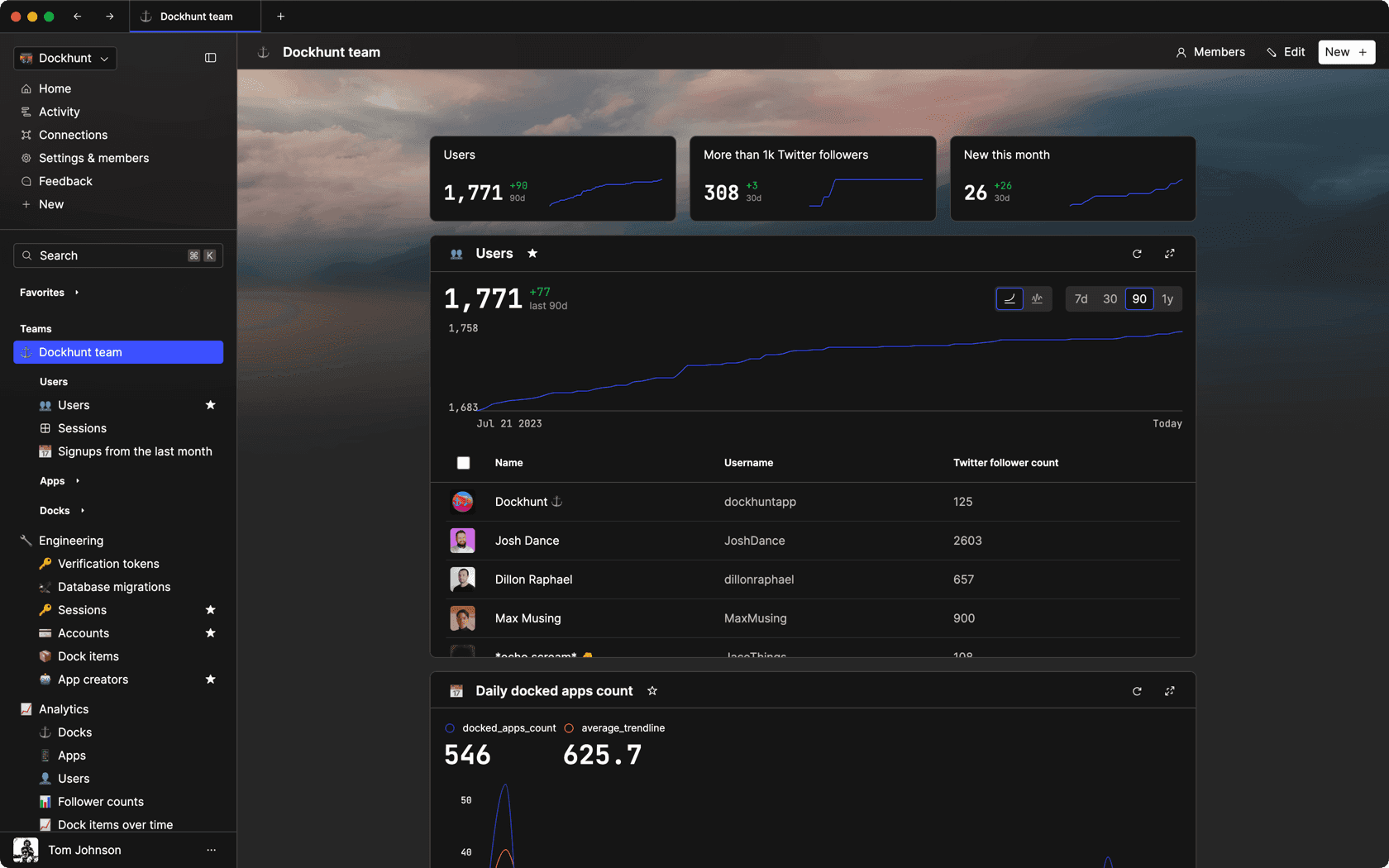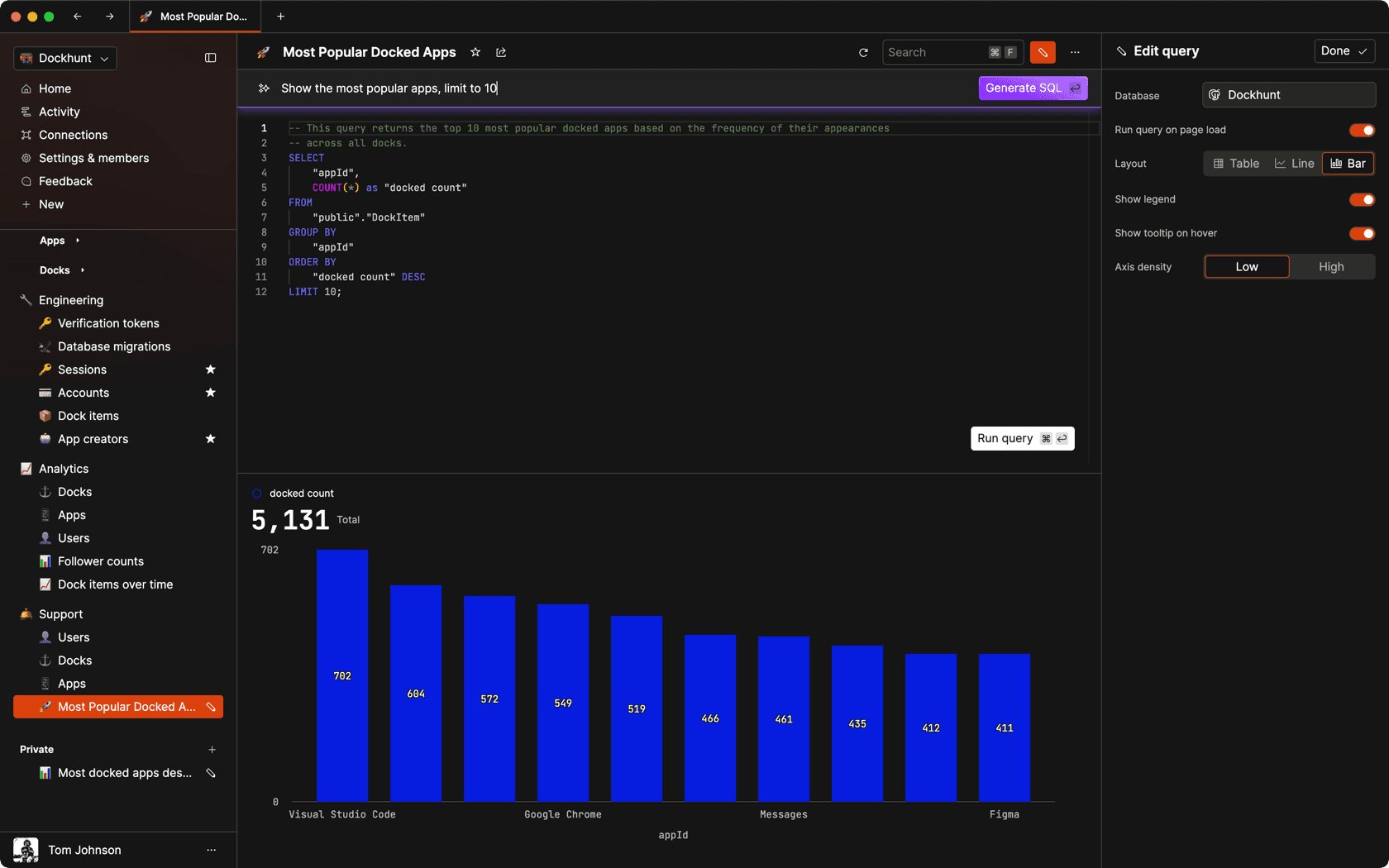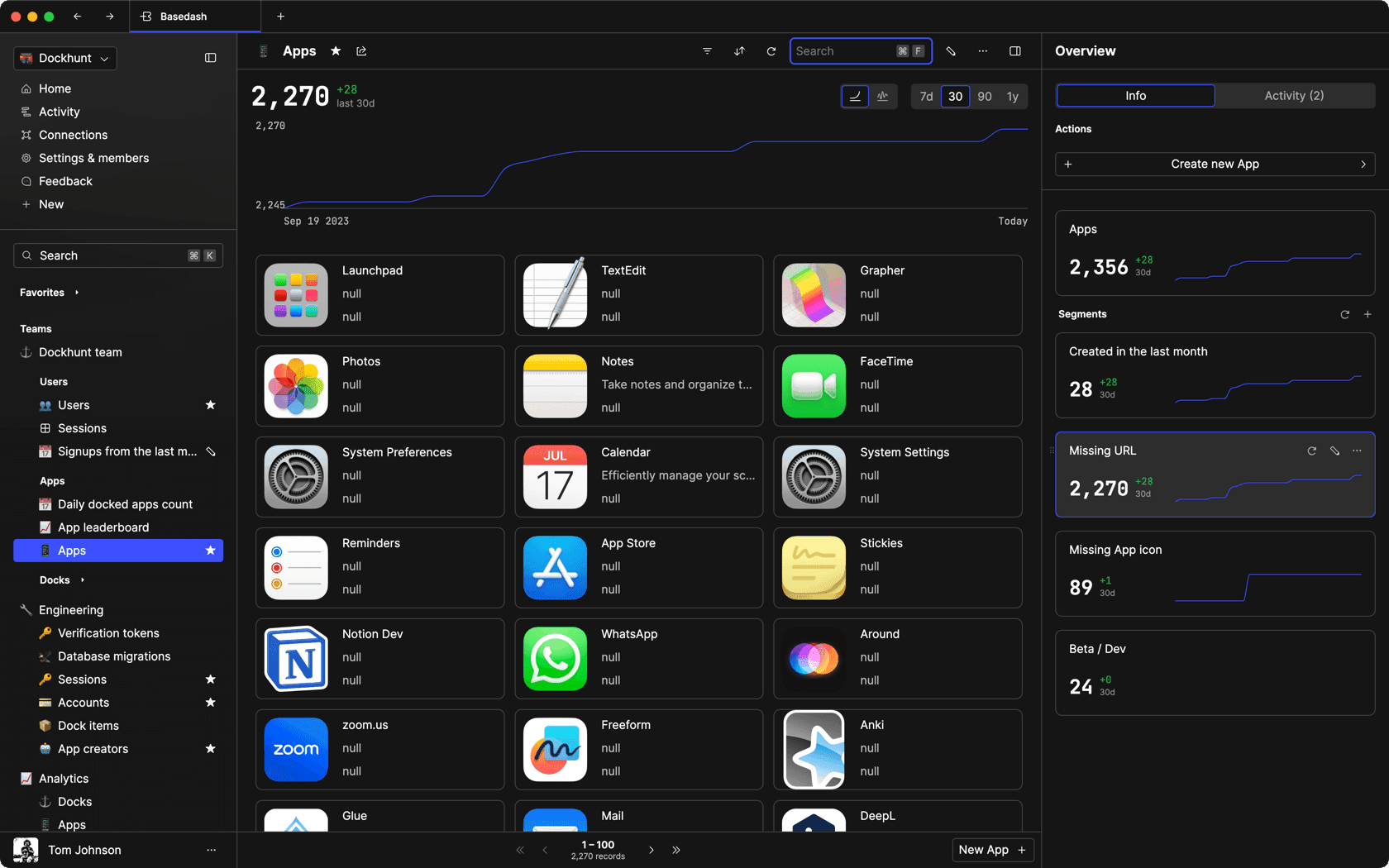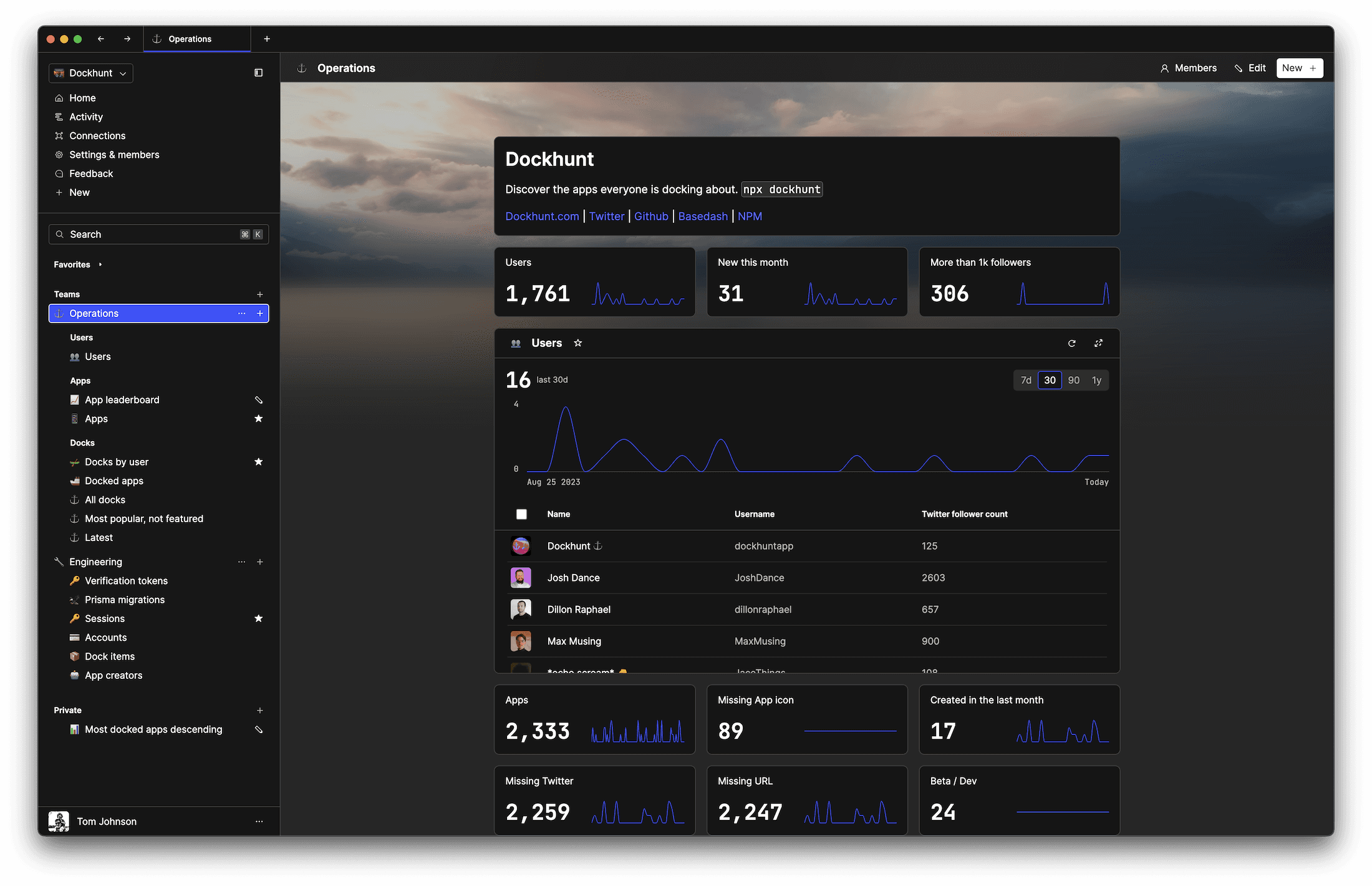# Percent in MySQL: An Overviewself.__wrap_n=self.__wrap_n||(self.CSS&&CSS.supports("text-wrap","balance")?1:2);self.__wrap_b=(e,r,t)=>{t=t||document.querySelector(`[data-br="\${e}"]`);let n=t.parentElement,a=s=>t.style.maxWidth=s+"px";t.style.maxWidth="";let o,i=n.clientWidth,l=n.clientHeight,c=i/2-.25,f=i+.5;if(i){for(a(c),c=Math.max(t.scrollWidth,c);c+1<f;)o=Math.round((c+f)/2),a(o),n.clientHeight===l?f=o:c=o;a(f*r+i*(1-r))}t.__wrap_o||typeof ResizeObserver<"u"&&(t.__wrap_o=new ResizeObserver(()=>{self.__wrap_b(0,+t.dataset.brr,t)})).observe(n)};self.__wrap_n!=1&&self.__wrap_b(":Rj0pm:",1)

November 10, 2023

Dealing with percentages in MySQL involves various operations like formatting data as a percent, calculating percentiles, and determining the top percentage of a dataset. This guide covers essential techniques and queries for handling percent-related operations in MySQL.

## Formatting as Percent

To format a value as a percent, multiply it by 100 and concatenate a '%' sign. This approach is useful for readability in results.

```SELECT CONCAT(ROUND(yourValue * 100, 2), '%') AS percent_formatted
FROM yourTable;```

## How to Get Ten Percent of a Value

Calculating 10% of a value in a MySQL query involves simply multiplying the value by 0.1.

```SELECT yourColumn * 0.1 AS ten_percent
FROM yourTable;```

## Ranking and Percent Rank

To rank rows in MySQL, use the `RANK()` or `DENSE_RANK()` function. For percent rank, divide the rank by the total number of rows.

```SET @total_rows = (SELECT COUNT(*) FROM yourTable);

SELECT
yourColumn,
RANK() OVER (ORDER BY yourColumn) AS rank,
RANK() OVER (ORDER BY yourColumn) / @total_rows AS percent_rank
FROM
yourTable;```

## Top Percent of Data

To select the top X percent of data, calculate the number of rows that represent the desired percentage and use it in a `LIMIT` clause.

```SET @percent = 10;
SET @limit = (SELECT ROUND(COUNT(*) * (@percent / 100)) FROM yourTable);

SELECT *
FROM yourTable
ORDER BY yourColumn DESC
LIMIT @limit;```

## Calculating Percentiles

Percentiles can be calculated using the `PERCENT_RANK()` function in window functions.

```SELECT
yourColumn,
PERCENT_RANK() OVER (ORDER BY yourColumn) AS percentile
FROM yourTable;```

### Dealing with Null Values in Percentage Calculations

When calculating percentages, handling null values is crucial to maintain the accuracy of your data. Neglecting null values can lead to incorrect calculations. Use `IFNULL` or `COALESCE` to handle these scenarios.

```-- Example: Calculating 10% of a value, treating nulls as zero
SELECT
yourColumn,
IFNULL(yourColumn, 0) * 0.1 AS ten_percent_handling_null
FROM yourTable;```

This query ensures that if `yourColumn` is null, it's treated as zero, preventing any miscalculations in your percentage results.

### Percentile Contiguous (PERCENTILE_CONT) for Specific Percentile Calculation

The `PERCENTILE_CONT` function in MySQL is used to compute the percentile for a given distribution of values. This function is particularly useful for finding median or any other specific percentile.

```-- Example: Calculating the median (50th percentile)
SELECT PERCENTILE_CONT(0.5) WITHIN GROUP (ORDER BY yourColumn) OVER () AS median
FROM yourTable;```

This query calculates the median value of `yourColumn` by finding the 50th percentile.

### Calculating Percent Increase or Decrease Between Two Values

Understanding how a value has changed over time often involves calculating the percentage increase or decrease. This is especially useful in financial or performance data analysis.

```-- Example: Calculating percent change between two values
SELECT
oldValue,
newValue,
((newValue - oldValue) / oldValue) * 100 AS percent_change
FROM yourTable;```

This query shows how much `newValue` has changed from `oldValue` in terms of percentage.

### Conditional Percentages Based on Criteria

In many scenarios, you might need to calculate percentages based on certain conditions. This is where conditional aggregation comes into play.

```-- Example: Calculating the percentage of rows that meet a specific condition
SELECT
COUNT(*) AS total_rows,
SUM(CASE WHEN condition THEN 1 ELSE 0 END) AS rows_meeting_condition,
SUM(CASE WHEN condition THEN 1 ELSE 0 END) / COUNT(*) * 100 AS conditional_percent
FROM yourTable;```

This query calculates what percentage of rows in `yourTable` meet the specified `condition`. It's a powerful way to get insights based on specific criteria within your data.

### Conclusion

This guide offers the foundation for dealing with various percent-related operations in MySQL, from formatting data for display to complex calculations involving percentiles and ranking. While these operations are core to data analysis, for more advanced data management solutions like creating admin panels or sharing SQL queries efficiently, consider exploring tools like Basedash.

# Basedash is the best MySQL admin panel

If you're building with MySQL, you need Basedash. It gives you an instantly generated admin panel to understand, query, build dashboards, edit, and share access to your data.

If you're building with MySQL, you need Basedash. It gives you an instantly generated admin panel to understand, query, build dashboards, edit, and share access to your data.

If you're building with MySQL, you need Basedash. It gives you an instantly generated admin panel to understand, query, build dashboards, edit, and share access to your data.### Dashboards and charts

Effortlessly make charts and create a space for your team to work together towards shared goals and metrics.

### SQL composer with AISOC II
Type 2 Certified

### Features

Proudly carbon-neutral.SOC II
Type 2 Certified

### Features

Proudly carbon-neutral.SOC II
Type 2 Certified